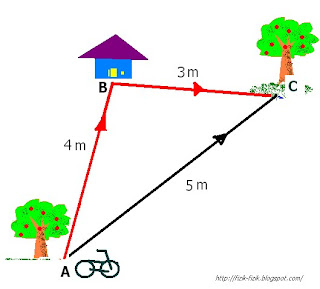## Monday, February 28, 2011

### Distance and displacementFigure shows a boy cycles from A to B, and then from B to C.

i ) Calculate the distance travelled by the boy and the displacement of the boy.

ii ) Calculate the speed and the velocity of the boy if the boy took 10 s to cycle from A to B and then to C.

iii) then the boy travelled back to A from C, calculate the total distance and displacement travelled by the boy.

Tips to answer this question :

Distance is the actual distance that was covered
Distance depends on the path, from point A --> B --> C in the figure.
Distance (symbol d) does not have a direction, so it is a scalar . It
always positive

Displacement (symbol s) is the shortest distance from the starting point to the endpoint.
Distance independent of path taken , from point A to C in the ﬁgure.
Displacement has direction and is therefore a vector. It can be positive or negative

Speed is rate of change of distance
. Speed is scalar quantity

Velocity is rate of change of displacement. Velocity vector quantity.

Distance
from A to B and then to is
3 m + 4 m
= 7 m

Displacement from A to B and then to C is 5 m.

Speed from A to B and then to C
7 m / 10 s
= 0.7 m/s

Velocity
from A to B and then to C is
5 m /10 s
= 0.5 m/s.

Distance from A-> B-> C -> B -> C is
3 m + 4 m + 3 m + 4 m
= 14 m

Displacement
from A-> B-> C -> B -> C is
5 m - 5 m
= 0 m
( Because the boy's location is the same as his starting location, we use negative sign to indicate opposite direction )

## Sunday, February 27, 2011

### Understanding scalar and vector quantities

In this unit you should be able to

1. define scalar and vector quantities
2. give examples of scalar and vector quantities.

Difference between scalar and vector quantities ,

1. Scalar quantities are quantities that have magnitude but no direction.

2. Vector quantities are quantities that have both magnitude and direction.

Examples of scalar and vector quantities:

 Scalar Quantities Vector Quantities Distance Displacement Speed Velocity Work Acceleration Area Force Length Momentum